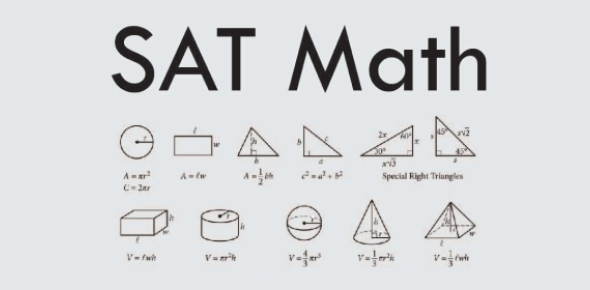# SAT Practice Test: Math Quiz! Trivia

18 Questions | Attempts: 2822
ShareSettingsAre you prepared to take the SAT’s? Do you suppose you can get past this quiz? Some tips to prepare for the SAT include registering for the test, get acclimated to the overall test structure and format. Then, become acquainted with the content and question styles. Zero in on any difficulties that you might have. Set a score goal. Make a study plan. Take this quiz and learn how to pass the SAT’s.

• 1.
If x + k = 12 and p(x + k) = 36, what is the value of p ?
• A.

3

• B.

4

• C.

6

• D.

9

• E.

12

• 2.
If 13 is added to one-half of a certain number the result is 37. What is the original number?
• A.

24

• B.

40

• C.

48

• D.

61

• E.

80

• 3.
In the figure above, the usual route from Town A to Town D is indicated by the solid line. The broken line indicates a detour route from B to C through E . Each line segment is labeled with its length in miles. How many more miles is the trip from Town A to Town D via the detour than via the usual route?
• A.

4

• B.

8

• C.

10

• D.

12

• E.

18

• 4.
Which of the following equations expresses y in terms of x for each of the four pairs of values shown in the table above?
• A.

Y = 5x + 7.5

• B.

Y = 5.5x + 2

• C.

Y = 5.5x + 7.5

• D.

Y = 7.5x

• E.

Y = 7.5x + 5.5

• 5.
In the figure above, point B lies on AC. If x and y are integers, which of the following is a possible value of x?
• A.

30

• B.

35

• C.

40

• D.

50

• E.

55

• 6.
The least and greatest numbers in a list of 7 real numbers are 2 and 20, respectively. The median of the list is 6, and the number 3 occurs most often in the list. Which of the following could be the average (arithmetic mean) of the numbers in the list?   I.7 II. 8.5 III. 10
• A.

I only

• B.

I and II only

• C.

I and III only

• D.

II and III only

• E.

I, II, and III

• 7.
In the XY-coordinate plane, how many points are a distance of 4 units from the origin?
• A.

One

• B.

Two

• C.

Three

• D.

Four

• E.

More than four

• 8.
The table above shows the number of consecutive nights that each of the five families stayed at a certain hotel during a 14-night period. If the Liu family’s stay did not overlap with the Benton family’s stay, which of the 14 nights could be a night on which the only one of the five families stayed at the hotel?
• A.

The 3rd

• B.

The 5th

• C.

The 6th

• D.

The 8th

• E.

The 10th

• 9.
If a cake is cut into thirds and each third is cut into fourths, how many pieces of cake are there?
• 10.
If y= h/x, where h is a constant, and if y = 3 when x = 4, what does y equal when x = 6?
• 11.
In the figure above, point B lies on side AC. If 55 < x < 60, what is one possible value of y ?
• 12.
The price of a certain item was \$10 in 1990 and it has gone up by \$2 per year since 1990. If this trend continues, in what year will the price be \$100?
• 13.
The figure above shows the graph of a quadratic function in the xy-plane. Of all the points (x, y) on the graph, for what value of x is the value of y greatest?
• 14.
The number n is a 2-digit number. When n is divided by 10, the remainder is 9, and when n is divided by 9, the remainder is 8. What is the value of n ?
• 15.
The area of the figure above is 9/4. What is the perimeter of the figure?
• 16.
If j is chosen at random from the set {4, 5, 6} and k is chosen at random from the set {10, 11, 12}, what is the probability that the product of j and k is divisible by 5?
• 17.
Tom and Alison are both salespeople. Tom’s weekly compensation consists of \$300 plus 20 percent of his sales. Alison’s weekly compensation consists of \$200 plus 25 percent of her sales. If they both had the same amount of sales and the same compensation for a particular week, what was that compensation, in dollars?
• 18.
Tx + 12y = -3 The equation above is the equation of a line in the XY-plane, and t is a constant. If the slope of the line is -10, what is the value of t?

## Related TopicsBack to top
×

Wait!
Here's an interesting quiz for you.